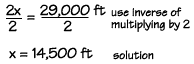Home    |    Teacher    |    Parents    |    Glossary    |    About UsMt. Everest in Nepal is the world's tallest mountain, about 29,000 ft. high. It is twice as high as Mount Whitney in California. How high is Mount Whitney?

We can write a multiplication equation to find the answer to problems like this. Our unknown number is the height of Mount Whitney. Let x represent this height. We know that 2x is the height of Mount Everest. We can write our equation like this:

2x = 29,000 ft

To solve this equation, we can use the inverse of multiplying by 2, which is dividing by 2.

If we divide the left side of the equation by 2, we will get x alone on the left. Remember, any operation done to one side must also be done to the other side, so we must also divide the right side by 2.We divide, and find that x is equal to 14,500 ft. This is very close to the actual height of Mount Whitney, which is 14,494 ft.

Mental Math

You don't always need to use the calculator or pencil and paper to solve equations. You can solve many of them mentally. Use your mental muscle on these problems! Come up with an answer on your own, before looking at the answers below.

1.  10x = 350
2.  (12)(5)n = 0
3.  6 + 6 = 3x
4.  19y = 1900

1.  x = 35 (Divide both sides by 10, an easy mental problem)
2.  n = 0 (Any number divided into 0 is 0.)
3.  x = 4 (6 + 6 = 12 and 12 ÷ 3 = 4)
4.  y = 100 (Divide both sides by 19, an easy mental problem)

 Homework Help | Algebra | Equations and InequalitiesEmail this page to a friendSearch·  Solving addition and     subtraction equations·  Multiplication equations·  Division equations·  Inequalities·  Formulas·  Two-step equations     and inequalitiesFirst Glance In Depth Examples WorkoutSolving multiplication equations